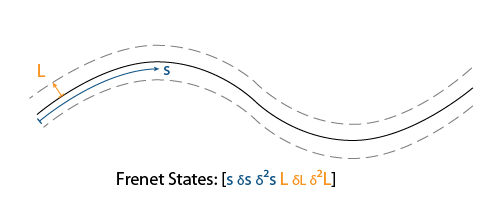# frenet2global

Convert Frenet states to global states

Since R2020b

## Syntax

``globalState = frenet2global(refPath,frenetState)``
``globalState = frenet2global(refPath,frenetState,latTimeDerivatives)``

## Description

example

````globalState = frenet2global(refPath,frenetState)` converts Frenet trajectory states to global states.```
````globalState = frenet2global(refPath,frenetState,latTimeDerivatives)` accepts `latTimeDerivatives` containing first and second-order derivatives of lateral deviation with respect to time and a flag indicating if the heading should flip during the conversion to global coordinates.```

## Examples

collapse all

Generate a reference path from a set of waypoints.

```waypoints = [0 0; 50 20; 100 0; 150 10]; refPath = referencePathFrenet(waypoints);```

Create a `trajectoryGeneratorFrenet` object from the reference path.

`connector = trajectoryGeneratorFrenet(refPath);`

Generate a five-second trajectory between the path origin and a point 30 meters down the path as Frenet states.

```initCartState = refPath.SegmentParameters(1,:); initFrenetState = global2frenet(refPath,initCartState); termFrenetState = initFrenetState + [30 zeros(1,5)]; frenetTraj = connect(connector,initFrenetState,termFrenetState,5);```

Convert the trajectory to the global states.

`globalTraj = frenet2global(refPath,frenetTraj.Trajectory);`

Display the reference path and the trajectory.

```show(refPath); axis equal hold on plot(globalTraj(:,1),globalTraj(:,2),'b')```

Specify global points and find the closest points on reference path.

```globalPoints = waypoints(2:end,:) + [20 -50]; nearestPathPoint = closestPoint(refPath,globalPoints);```

Display the global points and the closest points on reference path.

```plot(globalPoints(:,1),globalPoints(:,2),'r*','MarkerSize',10) plot(nearestPathPoint(:,1),nearestPathPoint(:,2),'b*','MarkerSize',10)```

Interpolate between the arc lengths of the first two closest points along the reference path.

```arclengths = linspace(nearestPathPoint(1,6),nearestPathPoint(2,6),10); pathStates = interpolate(refPath,arclengths);```

Display the interpolated path points.

```plot(pathStates(:,1),pathStates(:,2),'g') legend(["Waypoints","Reference Path","Trajectory to 30m",... "Global Points","Closest Points","Interpolated Path Points"])```## Input Arguments

collapse all

Reference path, specified as a `referencePathFrenet` object.

States in the Frenet coordinate frame, returned as a P-by-6 numeric matrix with rows of form `[S dS ddS L dL ddL]`, where `S` is the arc length and `L` is the perpendicular deviation from the direction of the reference path. Derivatives of `S` are relative to time. Derivatives of `L` are relative to the arc length, `S`. P is the total number of Frenet states.

Lateral time derivatives, specified as an N-by-3 matrix where each row is of the form [dL/dt ddL/dt^2 invertHeading] and N is the total number of points in `points`. Each row contains the 1st and 2nd order time derivatives of lateral deviation and a flag, invertHeading, which indicates whether the heading should be flipped when converting to global coordinates (`true`) or not (`false`).

Note

If defining `latTimeDerivatives` without the use of `global2frenet`, the following rules should be followed:

1. The invertHeading flag should be true when:

1. The vehicle is moving in reverse (speed is less than 0)

2. The vehicle is stationary (speed is equal to 0), and the vehicle is facing away from the path's tangent vector. i.e. cos(|tangentAngle(obj,S)-thetaExpected|) < 0

2. If 1b is true, then dL/dS must be negated.

## Output Arguments

collapse all

States in the global coordinate frame, specified as a P-by-6 numeric matrix with rows of form ```[x y theta kappa speed accel]```, where:

• x y and theta –– SE(2) state expressed in global coordinates, with x and y in meters and theta in radians.

• kappa –– Curvature, or inverse of the radius, in `m-1`.

• speed –– Speed in the `theta` direction in `m/s`.

• accel –– Acceleration in the `theta` direction in `m/s2`.

P is the total number of Global states.

collapse all

### Frenet Coordinate System Conversions

• The `global2frenet` object function finds a point ```[x y]p``` along the path that is closest to the xy-coordinate of the global state ```[x y theta kappa speed accel]g```. Since the reference path is C1 continuous (tangentially continuous), the distance will be shortest, where ```[x y]g-[x y]p``` is orthogonal to the tangent of the path.

where:

• x y and theta –– SE(2) state expressed in global coordinates, with x and y in meters and theta in radians.

• kappa –– Curvature, or inverse of the radius, in `m-1`.

• speed –– Speed in the theta direction in `m/s`.

• accel –– Acceleration in the theta direction in `m/s2`.

• The function then evaluates the full state of the path ```[x y theta kappa dkappa s]p```. This is equivalent to a moving reference frame located at ```[x y]p``` whose longitudinal axis points along `thetap` and lateral axis intersects with ```[x y]g``` (right-hand rule). The instantaneous motion of this frame is described by ```[kappa dkappa]p```.

where:

• x y and theta — SE(2) state expressed in global coordinates, with x and y in meters and theta in radians.

• kappa — Curvature, or inverse of the radius, in `m-1`.

• dkappa — Derivative of curvature with respect to arc length in `m-2`.

• s — Arc length, or distance along path from path origin, in meters.• The velocity and acceleration components of the global state act along the tangent defined by `thetag`, which itself evolves based on `kappag`. The function then maps the global position, velocity, and acceleration to the local Frenet frame and returns the Frenet state, ```[s ds dds L dL ddL]f```.

where:

• s — Arc length, or distance along path from path origin, in meters.

• L — Perpendicular deviation from the direction of the reference path, in meters.

• ds and dds — Derivatives of s relative to time.

• dL and ddL — Derivatives of L relative to the arc length, s.• The process for the `frenet2global` function is the inverse of the `global2frenet` function.

• The `frenet2global` function evaluates the path at the arc length of the Frenet state, `sf=sp`, returning ```[x y theta kappa dkappa s]p```.

• This point once again defines a reference frame whose axes and motion can be used to project the lateral or longitudinal deviations and their first and second-order derivatives back into the global frame.

## Version History

Introduced in R2020b